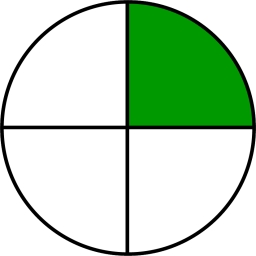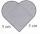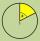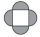# Quarter of a circle

Calculate the circumference of a quarter circle if its content is S = 314 cm2.

o =  71.3978 cm

### Step-by-step explanation:Did you find an error or inaccuracy? Feel free to write us. Thank you!#### You need to know the following knowledge to solve this word math problem:

We encourage you to watch this tutorial video on this math problem:

## Related math problems and questions:

• Four circles1) Calculate the circle radius if its area is 400 cm square 2) Calculate the radius of the circle whose circumference is 400 cm. 3) Calculate circle circumference if its area is 400 cm square 4) Calculate the circle's area if perimeter 400 cm.
• Math heartStylized heart shape created from a square with side 5 cm and two semicircles over his sides. Calculate the content area and its circumference.
• Quarter circleWhat is the radius of a circle inscribed in the quarter circle with a radius of 100 cm?
• CircleHow big is an area of a circle if its circumference is 51.2 cm?
• Area to perimeterCalculate circle circumference if its area is 254.34cm2
• Circle sectorThe circular sector with a central angle 160° has an area 452 cm2. Calculate its radius r.
• ArcCalculate span of the arc, which is part of a circle with diameter d = 20 m and its height is 6 m.
• Arc-sectorarc length = 17 cm area of sector = 55 cm2 arc angle = ? the radius of the sector = ?
• Right isoscelesCalculate area of the isosceles right triangle which perimeter is 26 cm.
• Square s3Calculate the diagonal of the square, where its area is 0.49 cm square. And also calculate its circumference.
• Cube surfce2volumeCalculate the volume of the cube if its surface is 150 cm2.
• DiscThe circumference of the disk is 78.5 cm. What is the circumference of the circular arc of 32° on the disc?
• SemicircleThe ornament consists of one square and four dark semicircles. The area of the square is 4 cm ^ 2. Find the area of one dark semicircle and round the result to hundreds.
• Arc and segmentCalculate the length of circular arc l, area of the circular arc S1 and area of circular segment S2. Radius of the circle is 11 and corresponding angle is (2)/(12) π.
• Circle - simpleCalculate the area of a circle in dm2 if its circumference is 31.4 cm.
• RectangleThe length of the rectangle is 12 cm greater than 3 times its width. What dimensions and area this rectangle has if ts circumference is 104 cm.
• Circular arcCalculate the length of the circular arc if the diameter d = 20cm and the angle alpha = 142 °